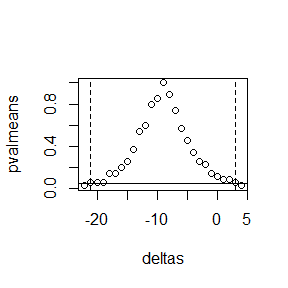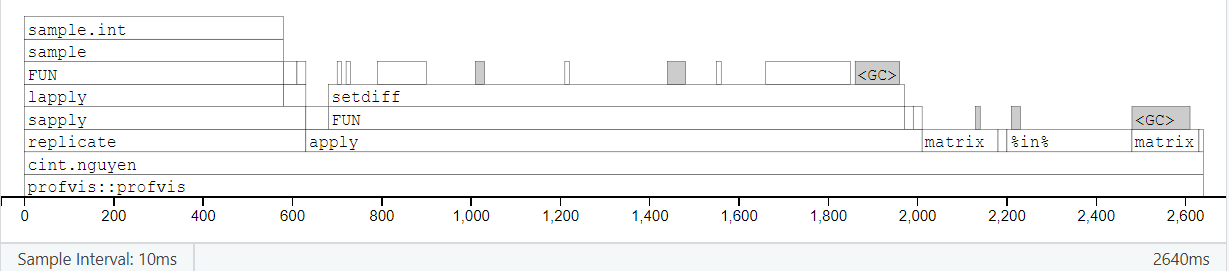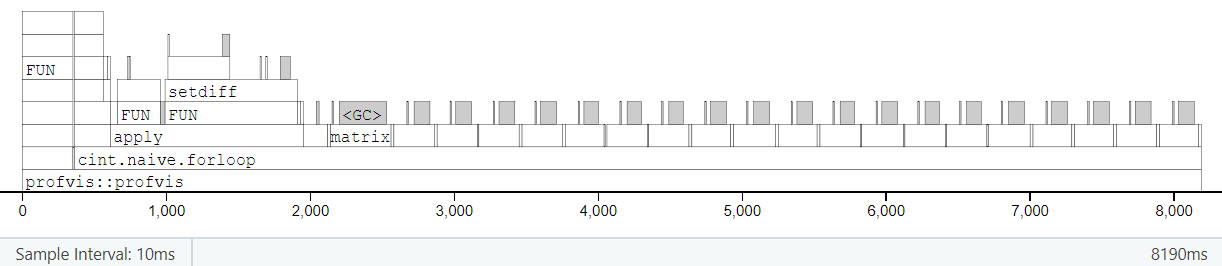# Comparing CIPerm with ‘naive’ approach

We run simple timing comparisons to show how our package’s approach with Nguyen (2009) compares against a “naive” grid-based search approach to confidence intervals from permutation methods.

# Defining streamlined functions

We can use CIPerm’s cint(dset(x, y)) directly, and it already wins against the naive for-loop or array-based approaches. But since dset and cint both have extra cruft built into them, for an even “fairer” comparison we recreate the core of cint(dset()) here without all the extra variables and without passing copies of dataframes.

First we re-define the internal function roundOrCeiling(), then we define a streamlined cint.nguyen():

#### Define a streamlined function for Nguyen approach ####

# 1. use round(siglevel*num), if siglevel*num is basically an integer,
#    to within the default numerical tolerance of all.equal();
# 2. or otherwise use ceiling(siglevel*num) instead.
roundOrCeiling <- function(x) {
ifelse(isTRUE(all.equal(round(x), x)), # is x==round(x) to numerical tolerance?
round(x),
ceiling(x))
}

cint.nguyen <- function(x, y, nmc = 10000, conf.level = 0.95) {
# Two-tailed CIs only, for now

sig <- 1 - conf.level

# Code copied/modified from within CIPerm::dset()
n <- length(x)
m <- length(y)
N <- n + m
num <- choose(N, n) # number of possible combinations
# Form a matrix where each column contains indices in new "group1" for that comb or perm
if(nmc == 0 | num <= nmc) { # take all possible combinations
dcombn1 <- utils::combn(1:N, n)
} else { # use Monte Carlo sample of permutations, not all possible combinations
dcombn1 <- replicate(nmc, sample(N, n))
dcombn1[,1] <- 1:n # force the 1st "combination" to be original data order
num <- nmc
}
# Form the equivalent matrix for indices in new "group2"
dcombn2 <- apply(dcombn1, 2, function(x) setdiff(1:N, x))

# Form the corresponding matrices of data values, not data indices
combined <- c(x, y)
group1_perm <- matrix(combined[dcombn1], nrow = n)
group2_perm <- matrix(combined[dcombn2], nrow = m)
# For each comb or perm, compute difference in group means, k, and w_{k,d}
diffmean <- colMeans(group1_perm) - colMeans(group2_perm)
k <- colSums(matrix(dcombn1 %in% ((n+1):N),
nrow = n))
wkd <- (diffmean - diffmean) / (k * (1/n + 1/m))

# Code copied/modified from within CIPerm::cint()
# Sort wkd values and find desired quantiles
w.i <- sort(wkd, decreasing = FALSE, na.last = FALSE)
siglevel <- (1 - conf.level)/2
index <- roundOrCeiling(siglevel*num) - 1
#   When dset's nmc leads us to use Monte Carlo sims,
#   we may get some permutations equivalent to orig data
#   i.e. we may get SEVERAL k=0 and therefore several w.i=NaN.
nk0 <- sum(k == 0)
# Start counting from (1+nk0)'th element of w.i
# (not the 1st, which will always be 'NaN' since k is 0)
LB <- w.i[1 + nk0 + index]
UB <- w.i[(num - index)]

CI <- c(LB, UB)
conf.achieved <- 1 - (2*(index+1) / num)
message(paste0("Achieved conf. level: 1-2*(", index+1, "/", num, ")"))
return(list(conf.int = CI,
conf.level.achieved = conf.achieved))
}

Next, we define an equivalent function to implement the “naive” approach:

• Choose a grid of possible values of delta = mu_X-mu_Y to try
• For each delta…
• subtract delta off of the x’s,
• and carry out a test of H_0: mu_X=mu_Y on the resulting data
• Our confidence interval consists of the range of delta values for which H_0 is NOT rejected
#### Define a function for "naive" approach with for-loop ####

cint.naive.forloop <- function(x, y, deltas,
nmc = 10000,
conf.level = 0.95) {
# Two-tailed CIs only, for now

sig <- 1 - conf.level
pvalmeans <- rep(1, length(deltas))

# Code copied/modified from within CIPerm::dset()
n <- length(x)
m <- length(y)
N <- n + m
num <- choose(N, n) # number of possible combinations
# Form a matrix where each column contains indices in new "group1" for that comb or perm
if(nmc == 0 | num <= nmc) { # take all possible combinations
dcombn1 <- utils::combn(1:N, n)
} else { # use Monte Carlo sample of permutations, not all possible combinations
dcombn1 <- replicate(nmc, sample(N, n))
dcombn1[,1] <- 1:n # force the 1st "combination" to be original data order
num <- nmc
}
# Form the equivalent matrix for indices in new "group2"
dcombn2 <- apply(dcombn1, 2, function(x) setdiff(1:N, x))

for(dd in 1:length(deltas)) {
xtmp <- x - deltas[dd]

# Code copied/modified from within CIPerm::dset()
combined <- c(xtmp, y)
# Form the corresponding matrices of data values, not data indices
group1_perm <- matrix(combined[dcombn1], nrow = n)
group2_perm <- matrix(combined[dcombn2], nrow = m)
# For each comb or perm, compute difference in group means
diffmean <- colMeans(group1_perm) - colMeans(group2_perm)

# Code copied/modified from within CIPerm::pval()
pvalmeans[dd] <- sum(abs(diffmean - mean(diffmean)) >= abs(diffmean - mean(diffmean)))/length(diffmean)
}
print( range(deltas[which(pvalmeans >= sig)]) )
return(list(cint = range(deltas[which(pvalmeans >= sig)]),
pvalmeans = pvalmeans,
deltas = deltas))
}

We also wrote another “naive” function that avoids for-loops and takes a “vectorized” approach instead. We created large arrays: each permutation requires a matrix, and the 3rd array dimension is over permutations. Then apply and colSums work very quickly to carry out all the per-permutations steps on this array at once. However, we found that unless datasets were trivially small, creating and storing the array took a lot of time and memory, and it ended up far slower than the for-loop approach. Consequently we do not report this function or its results here, although curious readers can find it hidden in the vignette’s .Rmd file.

# Speed tests

## Tiny dataset

When we compare the timings, cint.naive() will need to have a reasonable search grid for values of delta. On this problem, we happen to know the correct CI endpoints are the integers (-21, 3), so we “cheat” by using (-22):4 as the grid for cint.naive(). Of course in practice, if you had only the naive approach, you would probably have to try a wider grid, since you wouldn’t already know the answer.

#### Speed tests on Nguyen's tiny dataset ####

# Use 1st tiny dataset from Nguyen's paper
library(CIPerm)
x <- c(19, 22, 25, 26)
y <- c(23, 33, 40)

# Actual CIPerm package's approach:
system.time({
print( cint(dset(x, y), conf.level = 0.95, tail = "Two") )
})
#> Achieved conf. level: 1-2*(1/35)
#> $conf.int #>  -21 3 #> #>$conf.level.achieved
#>  0.9428571
#>    user  system elapsed
#>       0       0       0

# Streamlined version of CIPerm approach:
system.time({
print( cint.nguyen(x, y, conf.level = 0.95) )
})
#> Achieved conf. level: 1-2*(1/35)
#> $conf.int #>  -21 3 #> #>$conf.level.achieved
#>  0.9428571
#>    user  system elapsed
#>    0.05    0.00    0.04

# Naive approach with for-loops:
deltas <- ((-22):4)
system.time({
pvalmeans <- cint.naive.forloop(x, y, deltas)$pvalmeans }) #>  -21 3 #> user system elapsed #> 0.05 0.00 0.05 # Sanity check to debug cint.naive: # are the p-vals always higher when closer to middle of CI? # Are they always above 0.05 inside and below it outside CI? cbind(deltas, pvalmeans) #> deltas pvalmeans #> [1,] -22 0.02857143 #> [2,] -21 0.05714286 #> [3,] -20 0.05714286 #> [4,] -19 0.05714286 #> [5,] -18 0.14285714 #> [6,] -17 0.14285714 #> [7,] -16 0.20000000 #> [8,] -15 0.25714286 #> [9,] -14 0.37142857 #> [10,] -13 0.54285714 #> [11,] -12 0.60000000 #> [12,] -11 0.80000000 #> [13,] -10 0.85714286 #> [14,] -9 1.00000000 #> [15,] -8 0.88571429 #> [16,] -7 0.74285714 #> [17,] -6 0.57142857 #> [18,] -5 0.45714286 #> [19,] -4 0.34285714 #> [20,] -3 0.25714286 #> [21,] -2 0.22857143 #> [22,] -1 0.14285714 #> [23,] 0 0.11428571 #> [24,] 1 0.08571429 #> [25,] 2 0.08571429 #> [26,] 3 0.05714286 #> [27,] 4 0.02857143 plot(deltas, pvalmeans) abline(h = 0.05) abline(v = c(-21, 3), lty = 2)# Yes, it's as it should be :) Above we see timings for the original CIPerm::cint(dset)), its streamlined versioncint.nguyen(), and the naive equivalent cint.naive(). In the case of a tiny dataset like this, all 3 approaches take almost no time. So it can be a tossup as to which one is fastest on this example. ## Larger dataset Try a slightly larger dataset, where the total number of permutations is above the default nmc=10000 but still manageable on a laptop. Again, cint.naive() will need to have a reasonable search grid for values of delta. This time again we will set up a grid that just barely covers the correct endpoints from cint.nguyen(), and again we’ll try to keep it at around 20 to 25 grid points in all. Then we’ll try timing it again with a slightly finer grid. choose(18, 9) ## 48620 #>  48620 set.seed(20220528) x <- rnorm(9, mean = 0, sd = 1) y <- rnorm(9, mean = 1, sd = 1) # Actual CIPerm approach # (with nmc = 0, # so that we use ALL of the choose(N,n) combinations # instead of a MC sample of permutations) system.time({ print( cint(dset(x, y, nmc = 0), conf.level = 0.95, tail = "Two") ) }) #> Achieved conf. level: 1-2*(1216/48620) #>$conf.int
#>  -2.06178174  0.07679298
#>
#> $conf.level.achieved #>  0.9499794 #> user system elapsed #> 0.90 0.03 0.93 # Streamlined version of CIPerm approach: system.time({ print( cint.nguyen(x, y, nmc = 0, conf.level = 0.95) ) }) #> Achieved conf. level: 1-2*(1216/48620) #>$conf.int
#>  -2.06178174  0.07679298
#>
#> $conf.level.achieved #>  0.9499794 #> user system elapsed #> 0.76 0.04 0.81 # Coarser grid deltas <- ((-21):(1))/10 # grid steps of 0.1 # Naive with for-loops: system.time({ pvalmeans <- cint.naive.forloop(x, y, deltas, nmc = 0)$pvalmeans
})
#>  -2  0
#>    user  system elapsed
#>    1.54    0.29    1.90

# Finer grid
deltas <- ((-21*2):(1*2))/20 # grid steps of 0.05

# Naive with for-loops:
system.time({
pvalmeans <- cint.naive.forloop(x, y, deltas, nmc = 0)$pvalmeans }) #>  -2.05 0.05 #> user system elapsed #> 3.19 0.50 3.96 Now, CIPerm::cint(dset()) and the cint.nguyen approach take about the same amount of time, and they are noticeably faster than the cint.naive approach. (And even more so when we use a finer search grid.) That happens even with the “cheat” of using CIPerm first to find the right CI limits so that our naive search grid isn’t too wide, just stepping over the minimal required range with a reasonable grid-coarseness. (For the original data where CI = (-21, 3), we stepped from -22 to 4 in integers. For latest data where CI = (-2.06, 0.08), we stepped from -2.1 to 0.1 in units of 0.1 and then 0.05.) In practice there are probably cleverer ways for the naive method to choose grid discreteness and endpoints… but still, this seems like a more-than-fair chance for the naive approach. ## Largest dataset Try an even larger example, where we’re OK with a smallish total number of permutations (eg nmc=10000), but the dataset itself is “huge”, so that each individual permutation takes a longer time. We still don’t make it all that large, since we want this vignette to knit in a reasonable time. But we’ve seen similar results when we made even-larger datasets that took longer to run. Once again, for cint.naive() we will set up a search grid that just barely covers the correct endpoints from cint.nguyen(), and again we’ll try to keep it at around 20 to 25 grid points in all. #### Speed tests on much larger dataset #### set.seed(20220528) x <- rnorm(5e3, mean = 0, sd = 1) y <- rnorm(5e3, mean = 1, sd = 1) # Actual CIPerm approach # (with nmc = 2000 << choose(N,n), # so it only takes a MC sample of permutations # instead of running all possible combinations) system.time({ print( cint(dset(x, y, nmc = 2e3), conf.level = 0.95, tail = "Two") ) }) #> Achieved conf. level: 1-2*(50/2000) #>$conf.int
#>  -1.0227345 -0.9437299
#>
#> $conf.level.achieved #>  0.95 #> user system elapsed #> 8.76 1.63 11.23 # Streamlined version of CIPerm approach: system.time({ print( cint.nguyen(x, y, nmc = 2e3, conf.level = 0.95) ) }) #> Achieved conf. level: 1-2*(50/2000) #>$conf.int
#>  -1.0232198 -0.9435962
#>
#> $conf.level.achieved #>  0.95 #> user system elapsed #> 8.84 1.54 11.04 # Grid of around 20ish steps deltas <- ((-11*10):(-9*10))/100 # grid steps of 0.01 # Naive with for-loops: system.time({ pvalmeans <- cint.naive.forloop(x, y, deltas, nmc = 2e3)$pvalmeans
})
#>  -1.02 -0.95
#>    user  system elapsed
#>   13.83    6.75   21.71

Here we finally see that the overhead built into CIPerm::cint(dset()) makes it slightly slower than the streamlined cint.nguyen(). However, both are substantially faster than cint.naive()—they run in less than half the time of the naive approach.

# bench::mark() and profvis

The results above are from one run per method on each example dataset.

Using the last (“largest”) dataset, we also tried using bench::mark() to summarize the distribution of runtimes over many runs, as well as memory usage.
(Not actually run when the vignette knits, in order to avoid needing bench as a dependency.)

# bench::mark(cint.nguyen(x, y, nmc = 2e3),
#             cint.naive.forloop(x, y, deltas, nmc = 2e3),
#             check = FALSE, min_iterations = 10)
#> # A tibble: 2 × 13
#>   expression                                        min   median itr/sec mem_alloc gc/sec n_itr  n_gc total_time result memory                  time            gc
#>   <bch:expr>                                   <bch:tm> <bch:tm>     <dbl> <bch:byt>    <dbl> <int> <dbl>   <bch:tm> <list> <list>                  <list>          <list>
#> 1 cint.nguyen(x, y, nmc = 2000)                    2.5s    2.71s     0.364    1.58GB     2.92    10    80     27.44s <NULL> <Rprofmem [32,057 × 3]> <bench_tm > <tibble [10 × 3]>
#> 2 cint.naive.forloop(x, y, deltas, nmc = 2000)    7.87s    8.07s     0.120     7.4GB     4.64    10   388      1.39m <NULL> <Rprofmem [32,256 × 3]> <bench_tm > <tibble [10 × 3]>

Finally, we also tried using the profvis package to check what steps are taking the longest time.
(Again, not actually run when the vignette knits, in order to avoid needing profvis as a dependency; but screenshots are included below.)

# profvis::profvis(cint.nguyen(x, y, nmc = 2e3))
# profvis::profvis(cint.naive.forloop(x, y, deltas, nmc = 2e3))

For Nguyen’s method, the initial setup with combn() or sample() followed by apply(setdiff()) takes about 80% of the time, and the per-permutation calculations only take about 20% of the time:
profvis::profvis(cint.nguyen(x, y, nmc = 2e3))For the naive method, the initial setup is identical, and each round of per-permutation calculations is slightly faster than in Nguyen’s method… but because you have to repeat them many times (unless you already know the CI endpoints, in which case you wouldn’t need to run this at all!), they can add up to substantially more total time than for Nguyen’s method.
profvis::profvis(cint.naive.forloop(x, y, deltas, nmc = 2e3))